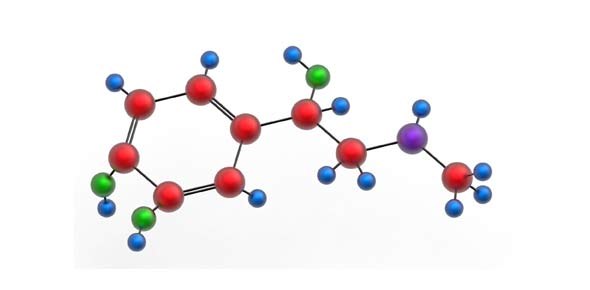# Mixtures And Compounds

9 Questions | Total Attempts: 62SettingsThis quiz is for 9th grade students

Related Topics
• 1.
Properties of matter that can be observed without changing a substance into a different one.
• A.

Chemical properties

• B.

Physical properties

• C.

Observable properties

• D.

Normal properties

• 2.
Groups of two or more atoms linked together
• A.

Ion

• B.

Molecule

• C.

Bond

• D.

Cation

• 3.
The compounds are composed of the same type of atoms linked together
• A.

True

• B.

False

• 4.
The sum of the atomic masses of all the atoms in the molecule is called
• A.

Atomic mass

• B.

Molecular weight

• C.

Molecular mass

• D.

Atomic weight

• 5.
The form colloid is one of the types of mixtures
• A.

True

• B.

False

• 6.
A uniform mixture in which the two substances are completely mixed
• A.

Colloid

• B.

Solution

• C.

Molecule

• D.

Uniform

• 7.
The most complete and informative of the chemical formulas
• A.

Molecular

• B.

Structural

• C.

Empirical

• D.

None

• 8.
H2O is an example of the following type of chemical formula
• A.

Molecular

• B.

Structural

• C.

Empirical

• D.

None

• 9.
Type of chemical formula that shows only the simplest ratio of atoms in a molecule
• A.

Molecular

• B.

Structural

• C.

Empirical

• D.

None